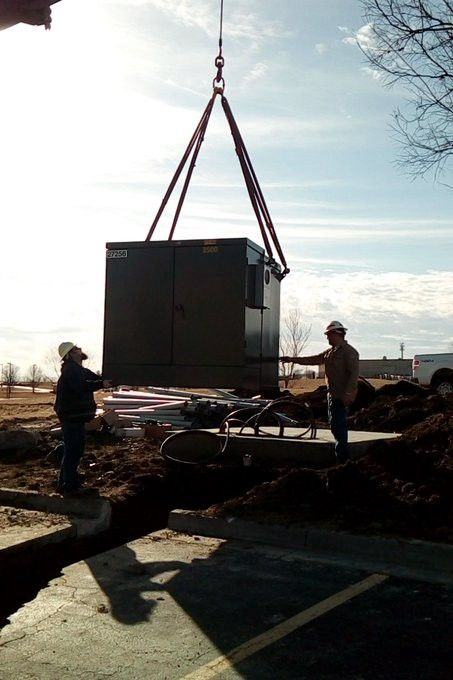Men installing a 2.5MW transformer outside a mining farm

# Bitcoin Mining Energy Consumption to Hashrate Guide

How many watts are needed per unit of hashrate? This guide breaks down how much power goes into producing bitcoin mining hashrate.

If you had a 3MW facility could you host 1,000 Bitmain Antminer T17+’s, and how much PH/second would that give you in hashing power?

Surprisingly, its not always the easiest to formulate in your head. So we put together a guide to help you through the process.

### Wattage (W)

A watt is a unit of power.

Power is a measure of the rate at which energy flows. Watts are a similar measurement to kilometers-per-hour as they indicate how fast electrons are travelling. One watt is equivalent to electricity flowing at a rate of one joule per second in the metric system.

Sometimes total watts are also referenced as Consumption or Reference\\ \\***Power on Wall *on platforms that are selling ASICs.

### KiloWatt-Hour (kWh)

kWh is a measure of energy (Involves Power &Time).

Energy is defined as the capacity to do work, such as hashing (mining). If you run a 3,000-watt Antminer T17+ for one hour, you’ve used 3,000 watt-hours, or 3.0 kWh. In other words, 3.0 kWh is the amount of energy you need to run a T17+ for an hour.

Usually the ASICs specs come with a +/- 10% and seem to get overclocked their reported energy consumption pretty easily. So if a T17+ says it consumes 3.0 it will likely go considerably higher. For simplicity lets assume no overclocking.

Specs from the Bitmain Antminer S17+ Released Dec 2019Almost every time you hear a miner talking about their cost they are referencing the electricity cost for a kWh. So if they say “I have 4 cent power” what they mean is they pay the electricity company (or hosting facility) 4 cents USD per kWh.

On a per month basis that would be:

(\$0.04 USD per kWh) x (24 hours/day) x (30.42 day/month ) = ~\$30 of cost per month per kWhSo to get the price of hosting a T17+ for a month just multiply its kWh (3.0) by that total (\$30) to get \$90 USD/Month

### MegaWatt (MW)

The term megawatt is usually used by a farm or colocation operator to describe how large their mining operations are.

A 1 MegaWatt farm, running at 100% capacity generates **1,000,000 **watts of power per hour (or 1,000 kW/h). So the plant produces 365 x 24 x 1 MegaWatt hours of total power a year.

However a 100% load factor is not feasible for an operation as the breakers and wires can’t usually take a continuous load. Around 80% capacity factor is more realistic.

So a 1MW farm can power up to 800kWh. If each S17+ is 3kWh then the farm can host 267 machines.Men installing a 2.5MW transformer outside a mining farm

A hash is the output of a hash function. Hash rate is the speed at which a computer is completing an operation in the cryptocurrency’s code. Hashrate is measured in hashes per second. A higher hashrate increases a miner’s opportunity of finding the next block and receiving the block reward.

If you join a PPS pool, your payout (i.e. Revenue) is dependant on your hashrate.

A terahash (TH) is equal to one trillion (1,000,000,000,000) hashes per second.

### Miner Efficiency

Now is when things get a bit thicker so put on your math hat.

The efficiency of an ASIC is one of the most important specs when considering a purchase. It is essentially how many shares (revenue) can the machine produce per unit of energy (cost). So this should give you a rough sense of profitability differences when comparing ASICs

The T17+ has a reference power efficiency on wall of** 44.0** J/TH (at @25°C). And if you remember our watt calculation from above 1 Watt = 1 Joule per second.

So to get to kWh per TH, the calculation for a T17+ is:

[44 Joules / (60 seconds x 60 minutes)] / (1000 Watts/kW) = 0.000012222 kWh / TH.So at an electricity price of \$0.04 kWH (referenced above) a miner would be paying \$0.0000004889 a TH.

And as a proof, if you multiply that cost by the stated TH/s of the ASIC (73) and by seconds in a month (60 x 60 x 24 x 30.42) and by the kWh of the machine (3.0) you should get back to the monthly hosting cost.

\$0.0000004889 a TH x (73TH/s) x (60 x 60 x 24 x 30.42) / (3.0kWh) = \$30#### Conclusion

So a 1MW farm can power 267 machines, each machine produce 73TH/s which will contribute a total of ~20PH.

### Hash Rate Calculations:

• 1 kilo hash per second is one thousand (1,000) hashes per second
• 1 mega hash per second is one million (1,000,000) hashes per second.
• 1 giga hash per second is one billion (1,000,000,000) hashes per second.
• 1 tera hash per second is one trillion (1,000,000,000,000) hashes per second.
• 1 peta hash per second is one quadrillion (1,000,000,000,000,000) hashes per second.
• 1 exa hash per second is one quintillion (1,000,000,000,000,000,000) hashes per second.

### Power Calculations:

• 1 kilowatt is one thousand (1,000) watts
• 1 megawatt is one million (1,000,000) watts
• 1 gigawatt is one billion (1,000,000,000) watts
Bitcoin Mining Guides

COO @ Luxor